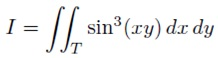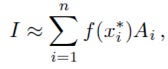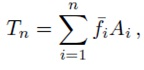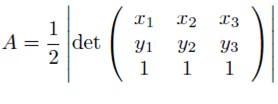#### Double Integrals for Non-rectangles

Double Integrals for Non-rectangles:

In the prior lecture we considered only integrals over rectangular regions. In reality regions of interest are rarely rectangles and therefore in this lecture we consider two strategies for evaluating integrals over other regions.

Redefining the function:

One strategy is to redefine the function therefore that it is zero outside the region of interest then integrate over a rectangle that includes the region.

For illustration suppose we need to approximate the value of:Where T is the triangle with corners at (0, 0), (1, 0) and (0, 2) afterwards we could let R be the rectangle [0, 1] × [0, 2] which contains the triangle T. See that the hypotenuse of the triangle has the equation 2x + y = 2. Afterwards make f(x) = sin3(xy) if 2x + y ≤ 2 and f(x) = 0 if 2x + y >2. In Mat lab we are able to make this function with the command:

> f = inline(’sin(x.*y).^3.*(2*x + y <= 2)’)

In this command <= is a logical command The expression in parentheses is then a logical statement and is given the value 1 if the statement is true and 0 if it is false. We are able to then integrate the modified f on [0, 1] × [0, 2] using the command:

> I = dblquad(f,0,1,0,2)

As another illustration suppose we need to integrate x2 exp(xy) inside the circle of radius 2 centered at (1, 2). The equation for this circle is (x − 1)2 + (y − 2)2 = 4. Note that the in the circle is (x−1)2 +(y −2)2 ≤ 4 and that the circle is contained in the rectangle [−1, 3]×[0, 4]. Therefore we can create the right function and integrate it by:

> f = inline(’x.^2.*exp(x.*y).*((x-1).^2 + (y-2).^2 <= 4)’)
> I = dblquad(f,-1,3,0,4)

Integration Based on Triangles:

The next approach to integrating over non-rectangular regions is based on subdividing the region into triangles. Such a subdivision is called the triangulation. On regions where the boundary comprises of line segments this can be done exactly. Yet on regions where the boundary contains curves this can be done approximately. This is a extremely important idea for several reasons the most important of which is that the finite elements method is based on it. Another reason this is significant is that often the values of f are not given by a formula however from data. For instance suppose you are surveying on a construction site as well as you want to know how much fill will be needed to bring the level up to the plan. You would carry on by taking elevations at numerous points across the site.

Nevertheless if the site is irregularly shaped or if there are obstacles on the site then you cannot make these measurements on an exact rectangular grid. In this case you can utilize triangles by connecting your points with triangles. Several software packages will even choose the triangles for you (Mat lab will do it using the command Delaunay).

The fundamental idea of integrals based on triangles is exactly the same as that for rectangles the integral is approximated by a sum where each term is a value times an area:where n is a number of triangles Aiis the area of the triangle and x* a point in the triangle.

Nevertheless rather than considering the value of f at just one point people habitually consider an average of values at several points. The mainly convenient of these is of course the corner points. We are able to represent this sum by:where f¯ is the average of f at the corners.

If a triangle has vertices (x1, y1), (x2, y2) and (x3, y3) the formula for area is:A function my three corners to calculate using the three corners method is given below.

Another idea would be to utilize the center point (centroid) of each triangle. If triangle has vertices (x1, y1), (x2, y2) and (x3, y3) then the centroid is given by the simple formulas:

x‾ =  (x1 + x2 + x3)/3 and y‾(y1 + y2 + y3)/3

function I = mythreecorners(f,V,T)
% Integrates a function based on a triangulation utilizing three corners
% Inputs: f -- the function to integrate as an inline
% V -- the vertices. Every row has the x and y coordinates of a vertex
% T -- the triangulation Every row gives the indices of three corners
% Output: the approximate integral
x = V(:,1); % extract x and y coordinates of all nodes
y = V(:,2);
I=0;
p = size(T,1);
for i = 1:p
x1 = x(T(i,1)); % find coordinates and area
x2 = x(T(i,2));
x3 = x(T(i,3));
y1 = y(T(i,1));
y2 = y(T(i,2));
y3 = y(T(i,3));
A = .5*abs(det([x1, x2, x3; y1, y2, y3; 1, 1, 1]));
z1 = f(x1,y1); % find values and average
z2 = f(x2,y2);
z3 = f(x3,y3);
zavg = (z1 + z2 + z3)/3;
I = I + zavg*A; % accumulate integral
End

Latest technology based Matlab Programming Online Tutoring Assistance

Tutors, at the www.tutorsglobe.com, take pledge to provide full satisfaction and assurance in Matlab Programming help via online tutoring. Students are getting 100% satisfaction by online tutors across the globe. Here you can get homework help for Matlab Programming, project ideas and tutorials. We provide email based Matlab Programming help. You can join us to ask queries 24x7 with live, experienced and qualified online tutors specialized in Matlab Programming. Through Online Tutoring, you would be able to complete your homework or assignments at your home. Tutors at the TutorsGlobe are committed to provide the best quality online tutoring assistance for Matlab Programming Homework help and assignment help services. They use their experience, as they have solved thousands of the Matlab Programming assignments, which may help you to solve your complex issues of Matlab Programming. TutorsGlobe assure for the best quality compliance to your homework. Compromise with quality is not in our dictionary. If we feel that we are not able to provide the homework help as per the deadline or given instruction by the student, we refund the money of the student without any delay.